# C - printf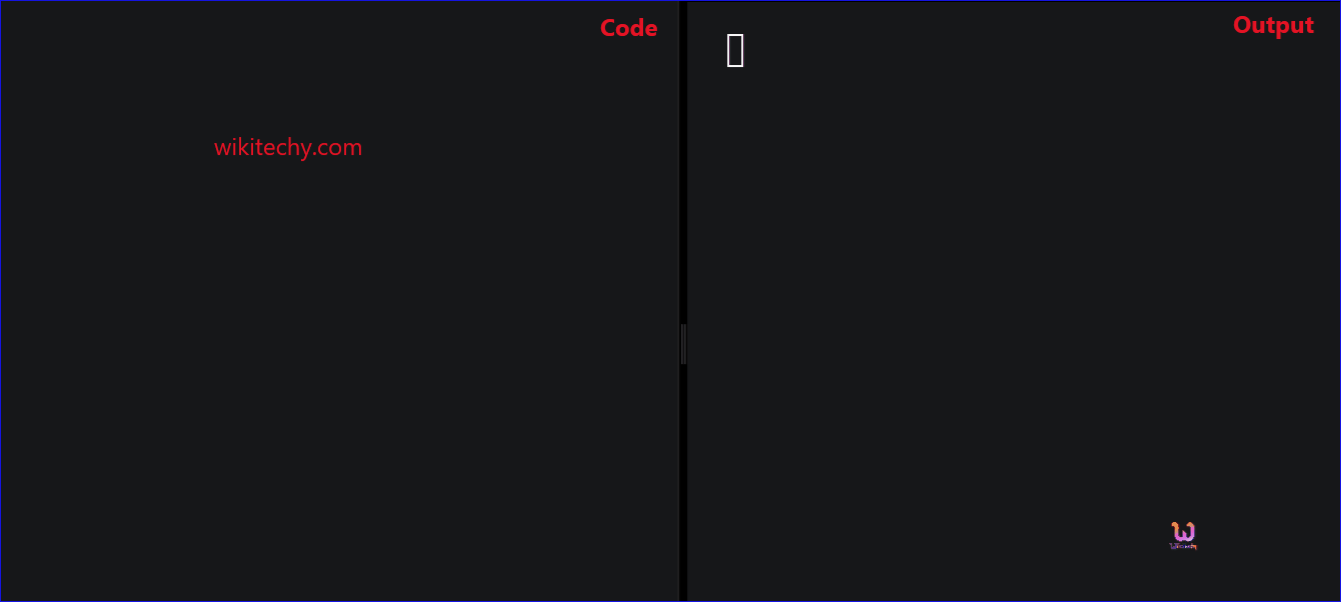Learn C - C tutorial - printf - C examples - C programs

## C - printf() Function - Definition and Usage

• The printf function is not the part of the C language, because there is no input or output defined in C language itself.
• In C , printf() function is used to print the “character, string, float, integer, octal and hexadecimal values” onto the output screen.
• We use printf() function with %d format specifier to display the value of an integer variable.
• Similarly, %c is used to display character, %f for float variable, %s for string variable, %lf for double and %x for hexadecimal variable.
• To generate a newline,we use “\n” in C printf() statement.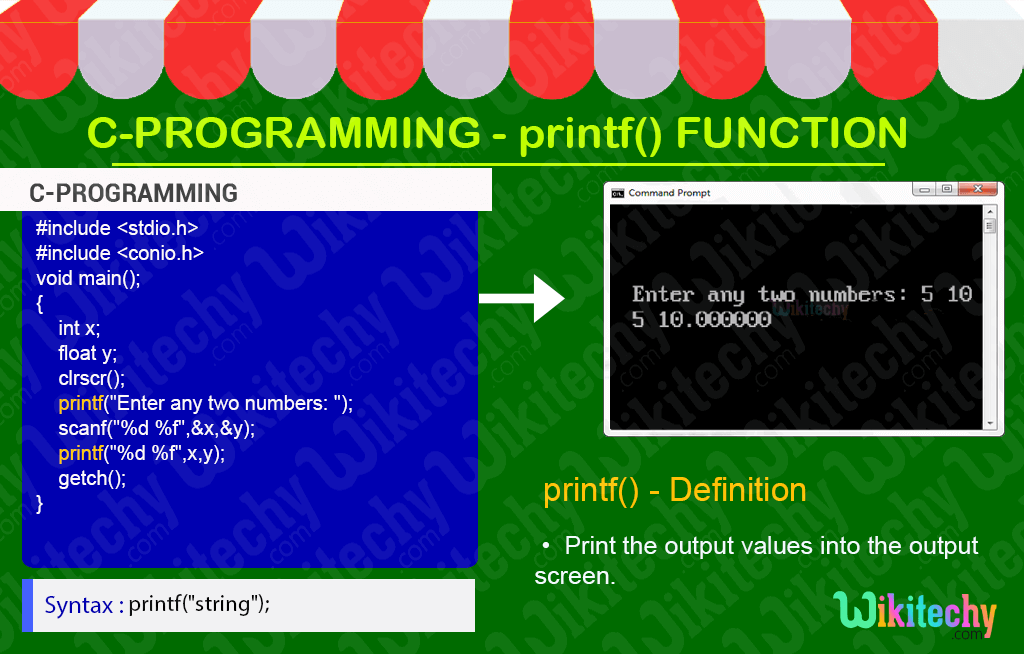## C Syntax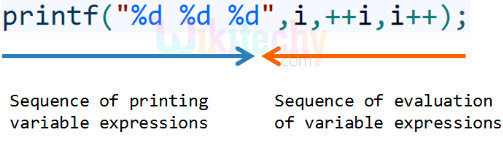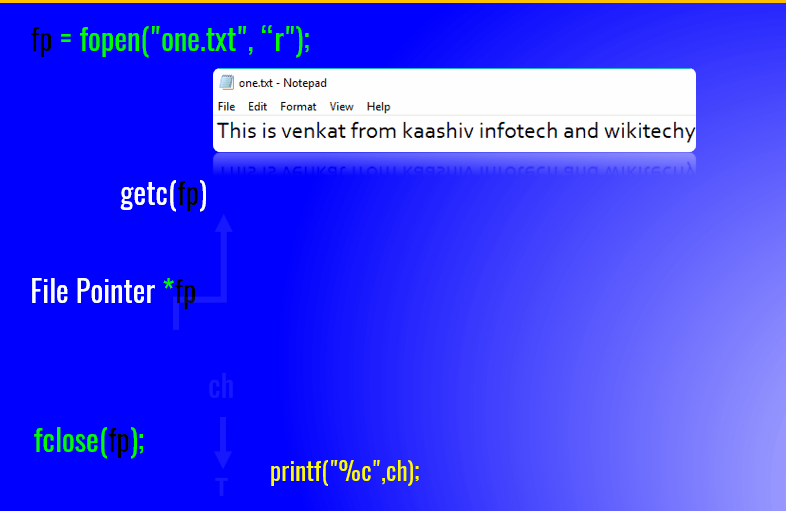## C Code - Explanation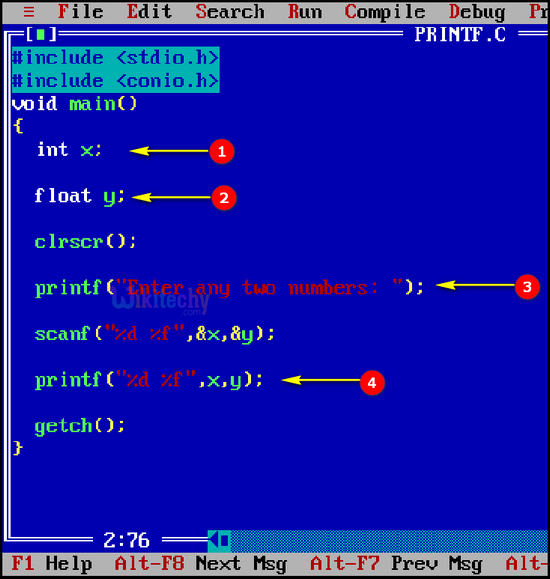1. In this example, “int x” specifies the integer value of the variable x.
2. Here, “float x” is specifying the float value of the variable y.
3. Here printf("Enter any two numbers: ") specifies the printf() function being used to print any two numbers in the output screen.
4. In this example, printf("%d %f",x,y) specifies that %d is an format specifier of integer value for the variable x. And %f is a float value of the variable y.

## Sample Output - Programming Examples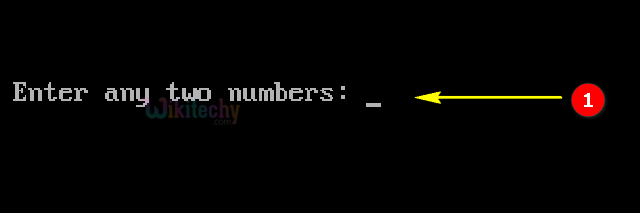1. Here in this output “Enter any two numbers: “ is represented in printf statement.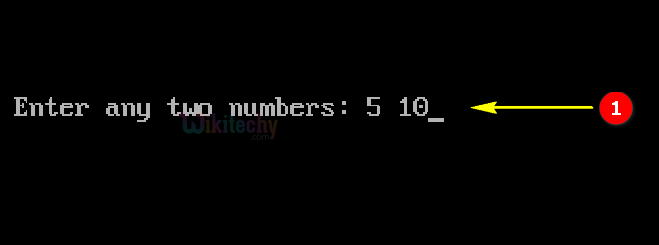1. Here in this output we are entering the values 5 and 10.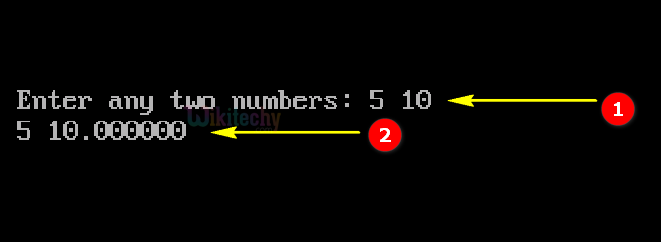1. Here in this output the entered values 5 and 10 are processed in the console window.
2. Here 5 is represented as integer value and 10.000000 as float value.